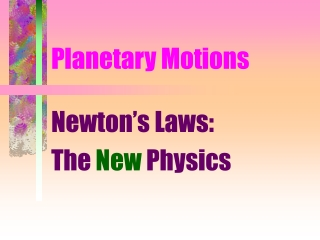DownloadDownload PresentationPlanetary Motions

# Planetary Motions

Télécharger la présentation## Planetary Motions

- - - - - - - - - - - - - - - - - - - - - - - - - - - E N D - - - - - - - - - - - - - - - - - - - - - - - - - - -
##### Presentation Transcript

1. Planetary Motions Newton’s Laws: The New Physics

2. Newton’s Laws • LAW 1: A body is in natural motion when it is at rest or when moving along at a constant speed along a straight line • Definition of natural motion

3. Newton’s Laws • LAW 2: A net force applied to a mass results in an acceleration OR • Acceleration is directly proportional to the net force and inversely proportional to the mass

4. Newton’s Laws • Speed is the rate of motion (km/s) • Velocity is speed with direction • Acceleration is a change in velocity (speed, direction, or both!) • Accelerations result from netforces (add them all!)

5. Newton’s Laws • Circular motion is accelerated motion (even if speed constant) • Why? Direction of motion is constantly changing! • Planetary motions are accelerated; implies a force

6. Newton’s Laws • LAW 3: Every force involves another equal but opposite force OR • Forces act in simultaneous pairs

7. Falling Bodies • Observation: All masses near surface of earth fall with same acceleration • Inference: Gravity acts so that the greater mass is pulled by a greater force

8. Gravitation • Interaction at a distance between masses (any size, shape) • More mass => more force (directly proportional to mass) • Greater distance => less force(inversely proportional to square of distance: inverse square law)

9. Newton’s Law of Gravitation • Force is proportional to(M1 x M2)/Distance2 • Outside of a body, acts as if all mass is concentrated at center • Weight is a force! • Predicts escape speed of one object leaving another (space travel!)

10. Newton’s Law of Gravitation • Results in Kepler’s laws (new version of 3rd; masses!) • Predicts/explains motions of planets with great precision • Physical basis of heliocentric model (earth’s rotation, revolution) • Powerful theory of gravitation

11. Newton’s Law of Gravitation • Predicts interactions of masses even outside solar system: a universal physical law! • Erased separation of earth and sky • Newton thought cosmos infinite; otherwise all masses would have already collapsed together